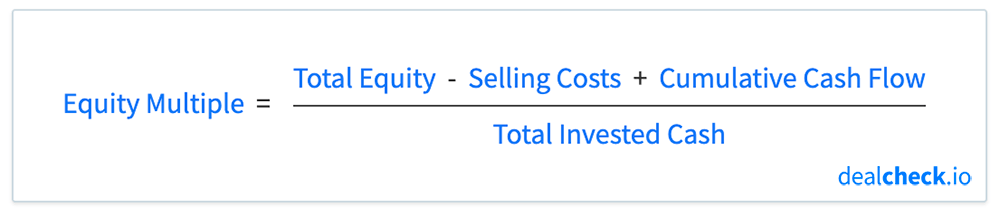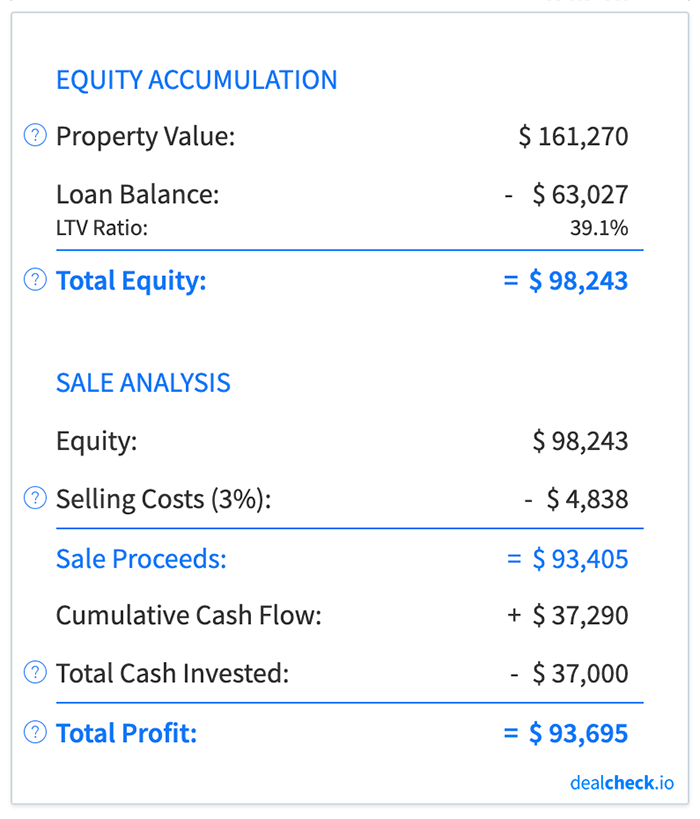The equity multiple is a measure of profitability and investment return commonly used by commercial real estate investors, although it can be used as a performance metric when analyzing and comparing any rental property or BRRRR.

Equity multiple takes into account the cumulative cash flow, equity accumulation, and loan paydown and shows the total cumulative return on your invested capital if you were to sell the property at a given point in time.

Unlike the return on investment (ROI), which uses a nearly identical formula, the equity multiple is expressed as a ratio instead of a percentage. It can be thought of as a “multiplier” of your total invested cash when considering your total profit from a property investment.

### The Equity Multiple Formula

The equity multiple can be calculated by taking the total profit from your investment, and dividing it by your total invested cash:In the formula above, your total profit is comprised of your equity (property value minus the loan balance), less the closing costs at the time of sale, as well as the cumulative cash flow received during the property holding period.

The total invested cash is simply all capital that was originally used to purchase and rehab the property. This usually includes the down payment on the loan (or the purchase price, if not using financing), purchase and closing costs, as well as rehab and repair costs.

### Equity Multiple Practical Example

Let’s assume that you purchased a rental property for \$100,000 with a 20% down payment (\$20,000) and paid \$2,000 in closing costs. The home also needed \$15,000 of rehab work to make it rent-ready.

You owned the property for 10 years before selling it for about \$161,000 and paying \$4,800 in closing costs in the process. Throughout the 10 years, you also collected just over \$37,000 of cash flow from this property.

First, let’s calculate how much capital you spent to purchase and rehab this property – your total invested cash:

Total Invested Cash = Down Payment + Closing Costs + Rehab Costs = \$20,000 + \$2,000 + \$15,000 = \$37,000

Next, let’s calculate how much money you will receive when you sell this property after 10 years – your sale proceeds:

Sale Proceeds = Sale Price – Loan Balance – Closing Costs = \$161,270 – \$63,027 – \$4,838 = \$93,405Finally, let’s calculate your equity multiple after 10 years of ownership:

Equity Multiple = Sale Proceeds + Cumulative Cash Flow / Total Invested Cash = \$93,405 + \$37,290 / \$37,000 = 3.53x

So what does this number tell you? Generally, an equity multiple less than 1.0x means that you will lose money on a particular investment – in other words, your total profit will be negative. On the other hand, an equity multiple greater than 1.0x means that you will make at least some profit after selling the property.

In the example above, at the end of the 10 year holding period, you will achieve an equity multiple of 3.53x. This means that for every \$1 invested, you will receive \$3.50 back when you sell the property at the end of 10 years. Note, that the \$3.50 also includes your original investment, so your actual net profit will be \$2.50 on the dollar.

### Equity Multiple vs. ROI

The equity multiple and the return on investment (ROI) represent the same profitability metric and return on your investment. The only difference between the two is that the ROI shows the return as a percentage, while the equity multiple shows it as a ratio or “multiple”.

In the example above, we calculated the equity multiple for the property to be 3.53x. If we were to calculate the return on investment for the same property, we would get the same figure, but expressed as a percentage:

ROI = Sale Proceeds + Cumulative Cash Flow – Total Invested Cash / Total Invested Cash = \$93,405 + \$37,290 – \$37,000 / \$37,000 = 253%

Going in reverse, if we were to express the 253% return on investment as a multiple instead of a percentage, we would calculate it as 1 + 253% / 100 = 3.53x. This is identical to the equity multiple we calculated earlier.### Equity Multiple vs. IRR

Another popular measure of investment return that is often calculated and displayed along with the equity multiple is the internal rate of return, abbreviated as IRR.

While the equity multiple shows the total cumulative return on your invested capital, the IRR shows an average annualized return instead. The IRR also takes into account the time value of money, while the equity multiple does not.

So when should you focus on one of these metrics versus the other?

Since the equity multiple shows the total cumulative return, it is good for estimating the total return (and profit) you will receive from your investment after a certain period of time. While it doesn’t take into account how long it took to realize this return, it is a good measure of how much profit you will get from your investment.

On the other hand, the IRR does not show your total return, but instead an average annualized return over the entire holding period. Since it takes the time value of money into account, it can help you understand how the length of the holding period will affect your return.

Because these metrics show different returns, it is often helpful to consider both the equity multiple and the IRR together when evaluating and comparing investment properties. You can use the equity multiple to compare the total returns on your capital, and IRR to compare the average annualized returns.

### Calculate Equity Multiple and Dozens of Other Metrics in Seconds

The DealCheck property analysis app makes it easy to calculate the equity multiple, along with dozens of other property analysis metrics for commercial and residential properties in seconds.